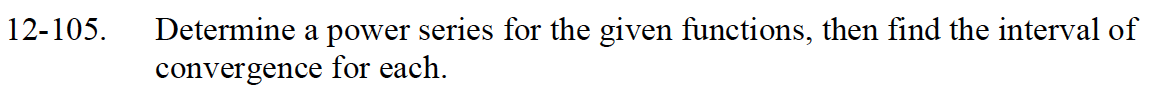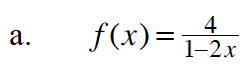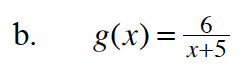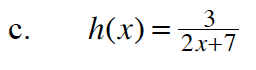### Home > CALC > Chapter Ch12 > Lesson 12.3.1 > Problem12-105

12-105.$f(x)=4\Big(\frac{1}{1-(2x)}\Big)$

$p(x)=4(1+(2x)+(2x)^2+(2x)^3+...)$

$p(x)=4\sum_{n=0}^\infty (2x)^n$

$\lim_{n\to\infty}\Big|\frac{2^{n+1}x^{n+1}}{2^nx^n}\Big|$

$2|x|<1$$g(x)=6\Big(\frac{1}{5(1-(-x/5))}\Big)$

$g(x)=\frac{6}{5}\Big(\frac{1}{1-(-x/5)}\Big)$

Now follow the steps outlined in part (a).$h(x)=3\Big(\frac{1}{7(1-(-2x/7)}\Big)$

Now follow the steps outlined in parts (b) and (c).